# What is Object Diagram?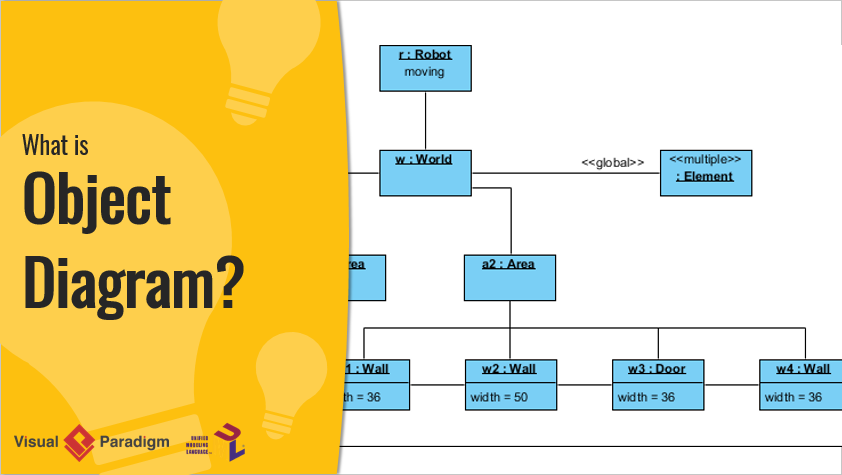Object is an instance of a class in a particular moment in runtime that can have its own state and data values. Likewise a static UML object diagram is an instance of a class diagram; it shows a snapshot of the detailed state of a system at a point in time, thus an object diagram encompasses objects and their relationships which may be considered a special case of a class diagram or a communication diagram.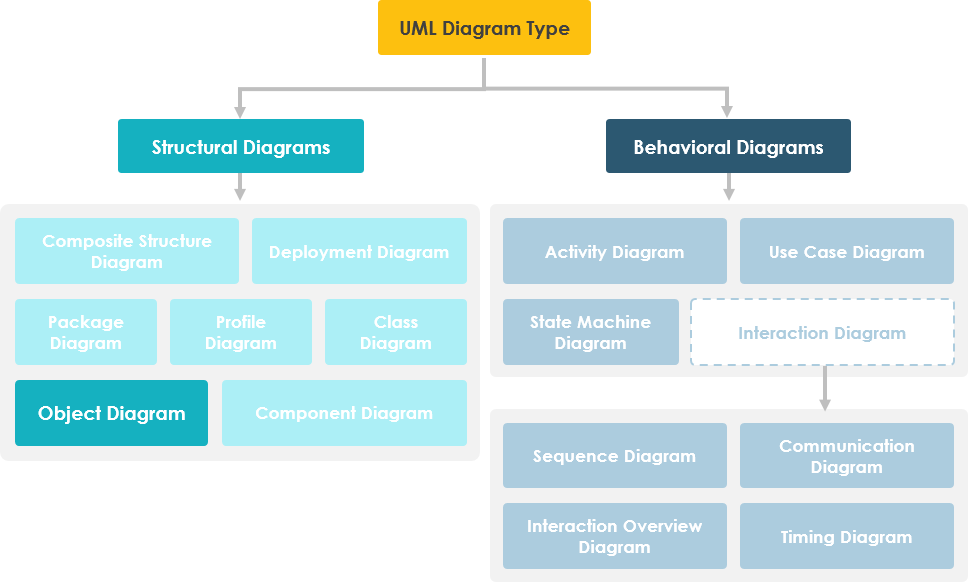# Learn UML Faster, Better and Easier

Are you looking for a Free UML tool for learning UML faster, easier and quicker? Visual Paradigm Community Edition is a UML software that supports all UML diagram types. It is an international award-winning UML modeler, and yet it is easy-to-use, intuitive & completely free.

## Purpose of Object Diagram

The use of object diagrams is fairly limited, mainly to show examples of data structures.

• During the analysis phase of a project, you might create a class diagram to describe the structure of a system and then create a set of object diagrams as test cases to verify the accuracy and completeness of the class diagram.
• Before you create a class diagram, you might create an object diagram to discover facts about specific model elements and their links, or to illustrate specific examples of the classifiers that are required.

## Object Diagram at a Glance

An object diagram shows this relation between the instantiated classes and the defined class, and the relation between these objects in the system. They are be useful to explain smaller portions of your system, when your system class diagram is very complex, and also sometimes modeling recursive relationship in diagram.

The best way to illustrate what an object diagram look like is to show the object diagram derived from the corresponding class diagram.

The following Order Management System shows their relationships. This small class diagram shows that a university Department can contain lots of other Departments and the object diagram below instantiates the class diagram, replacing it by a concrete example.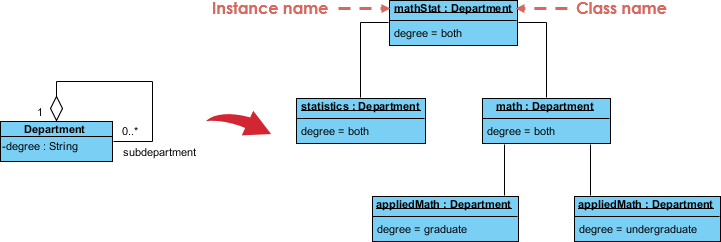## Class to Object Diagram Example - Order System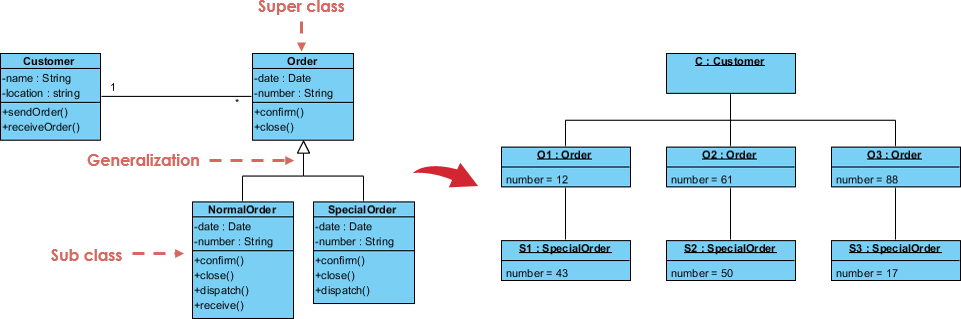## Basic Object Diagram Symbols and Notations

 Object Names: Every object is actually symbolized like a rectangle, that offers the name from the object and its class underlined as well as divided with a colon.Object Attributes: Similar to classes, you are able to list object attributes inside a separate compartment. However, unlike classes, object attributes should have values assigned for them.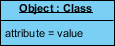Links: Links tend to be instances associated with associations. You can draw a link while using the lines utilized in class diagrams.## Class Diagram vs. Object Diagram

In UML, object diagrams provide a snapshot of the instances in a system and the relationships between the instances. By instantiating the model elements in a class diagram, you can explore the behavior of a system at a point in time.

• An object diagram is a UML structural diagram that shows the instances of the classifiers in models.
• Object diagrams use notation that is similar to that used in class diagrams.
• Class diagrams show the actual classifiers and their relationships in a system
• Object diagrams show specific instances of those classifiers and the links between those instances at a point in time.
• You can create object diagrams by instantiating the classifiers in class, deployment, component, and use-case diagrams.

## Object Diagrams - Learn by Examples

### Object Diagram Example I - Company Structure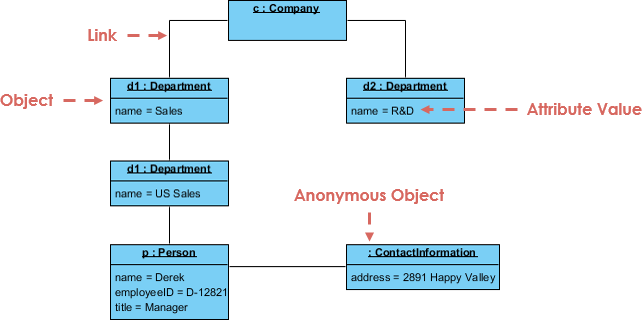### Object Diagram Example II - POS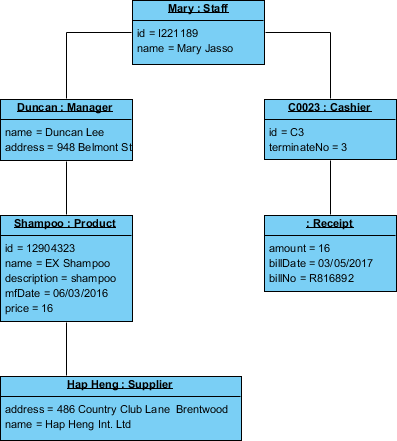### Object Diagram Example III - Writer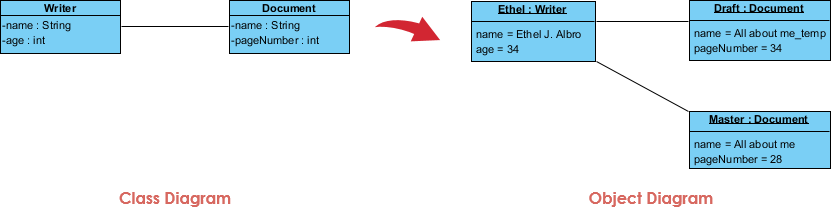## Steps for Modeling Object Structures

A communication diagram (called collaboration in previous version of UML) without messages is also known as an object diagram, and the relationships between objects are called links. An object diagram must be a valid instantiation of a static class diagram. Objects must have classes and links between objects must be instances of associations between classes. This can be used as a quick consistency check. To do this we can develop an object diagram by using the following steps:

• Identify the mechanism you'd like to model. A mechanism represents some functions or behaviors of the part of the system you are modeling that results from the interaction of a society of classes, interfaces, and other things.
• For each mechanism, identify the classes, interfaces, and other elements that participate in this collaboration; identify the relationships among these things, as well.
• Consider one scenario that walks through this mechanism. Freeze that scenario at a moment in time, and render each object that participates in the mechanism.
• Expose the state and attribute values of each such object, as necessary, to understand the scenario.

## Object Diagram Example IV - Robot Moving Behavior

Similarly, expose the links among these objects, representing instances of associations among them.

As this figure indicates, one object represents the robot itself (r, an instance of Robot), and r is currently in the state marked moving. This object has a link to w, an instance of World, which represents an abstraction of the robot's world model. This object has a link to a multiple objects that consists of instances of Element, which represent entities that the robot has identified but not yet assigned in its world view. These elements are marked as part of the robot's global state.

At this moment in time, w is linked to two instances of Area. One of them (a2) is shown with its own links to three Wall and one Door object. Each of these walls is marked with its current width, and each is shown linked to its neighboring walls. As this object diagram suggests, the robot has recognized this enclosed area, which has walls on three sides and a door on the fourth.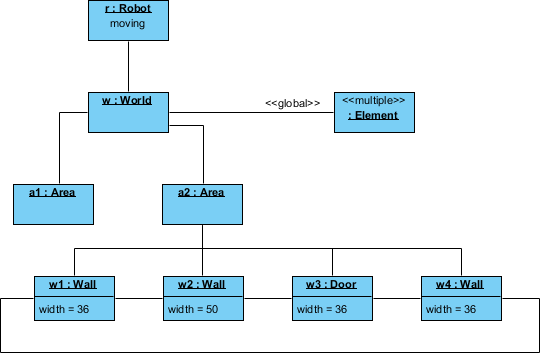## Object Diagram Example V - Deriving an Object Structure Similar to Communication Diagram

Besides showing the objects' state at a particular point in time, an object diagram can also be used to represent the occurrences of interactions between classes in runtime. The result looks a bit like a communication diagram. The figure below provides an example of such a class diagram and its corresponding object diagram:# Try to Draw UML Object Diagram Now

You've learned what an Object Diagram is and how to draw a Object Diagram. It's time to draw a Object Diagram of your own. Get Visual Paradigm Community Edition, a free UML software, and create your own Object Diagram with the free Object Diagram tool. It's easy-to-use and intuitive.